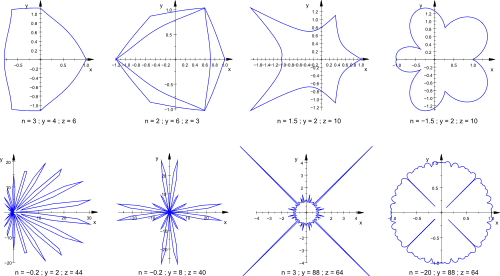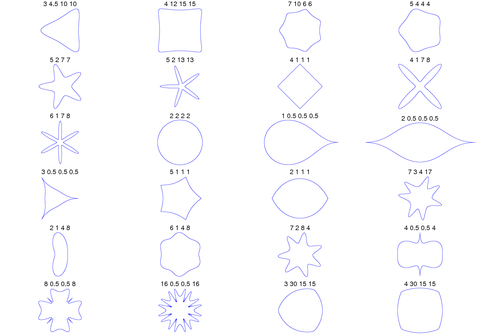# Superformula

The superformula is a generalization of the superellipse and was proposed by Johan Gielis around 2000. Gielis suggested that the formula can be used to describe many complex shapes and curves that are found in nature. Gielis holds a patent related to the synthesis of patterns generated by the superformula.

In polar coordinates, withthe radius andthe angle, the superformula is:By choosing different values for the parametersanddifferent shapes can be generated.

The formula was obtained by generalizing the superellipse, named and popularized by Piet Hein, a Danish mathematician.

## Extension to higher dimensions

It is possible to extend the formula to 3, 4, or n dimensions, by means of the spherical product of superformulas. For example, the 3D parametric surface is obtained by multiplying two superformulas r1 and r2. The coordinates are defined by the relations:where(latitude) varies between −π/2 and π/2 and θ (longitude) between −π and π.

## Generalization

The superformula can be generalized by replacing the parameterwith y andwith z:This allows the creation of rotationally asymmetric and nested structures. In the following examples a, b,andare 1:## PlotsA GNU Octave program for generating these figures:

function sf2d(n, a)
u = [0:.001:2 * pi];
raux = abs(1 / a(1) .* abs(cos(n(1) * u / 4))) .^ n(3) + abs(1 / a(2) .* abs(sin(n(1) * u / 4))) .^ n(4);
r = abs(raux) .^ (- 1 / n(2));
x = r .* cos(u);
y = r .* sin(u);
plot(x, y);
end


3D superformula: a = b = 1;== m, n1, n2 and n3 are shown in the pictures.

A GNU Octave program for generating these figures:

function sf3d(n, a)
u = [- pi:.05:pi];
v = [- pi / 2:.05:pi / 2];
nu = length(u);
nv = length(v);
for i = 1:nu
for j = 1:nv
raux1 = abs(1 / a(1) * abs(cos(n(1) .* u(i) / 4))) .^ n(3) + abs(1 / a(2) * abs(sin(n(1) * u(i) / 4))) .^ n(4);
r1 = abs(raux1) .^ (- 1 / n(2));
raux2 = abs(1 / a(1) * abs(cos(n(1) * v(j) / 4))) .^ n(3) + abs(1 / a(2) * abs(sin(n(1) * v(j) / 4))) .^ n(4);
r2 = abs(raux2) .^ (- 1 / n(2));
x(i, j) = r1 * cos(u(i)) * r2 * cos(v(j));
y(i, j) = r1 * sin(u(i)) * r2 * cos(v(j));
z(i, j) = r2 * sin(v(j));
endfor;
endfor;
mesh(x, y, z);
endfunction;

1. Gielis, Johan (2003), "A generic geometric transformation that unifies a wide range of natural and abstract shapes", American Journal of Botany, 90 (3): 333–338, doi:10.3732/ajb.90.3.333, ISSN 0002-9122
2. EP patent 1177529, Gielis, Johan, "Method and apparatus for synthesizing patterns", issued 2005-02-02Wikimedia Commons has media related to Superformula.